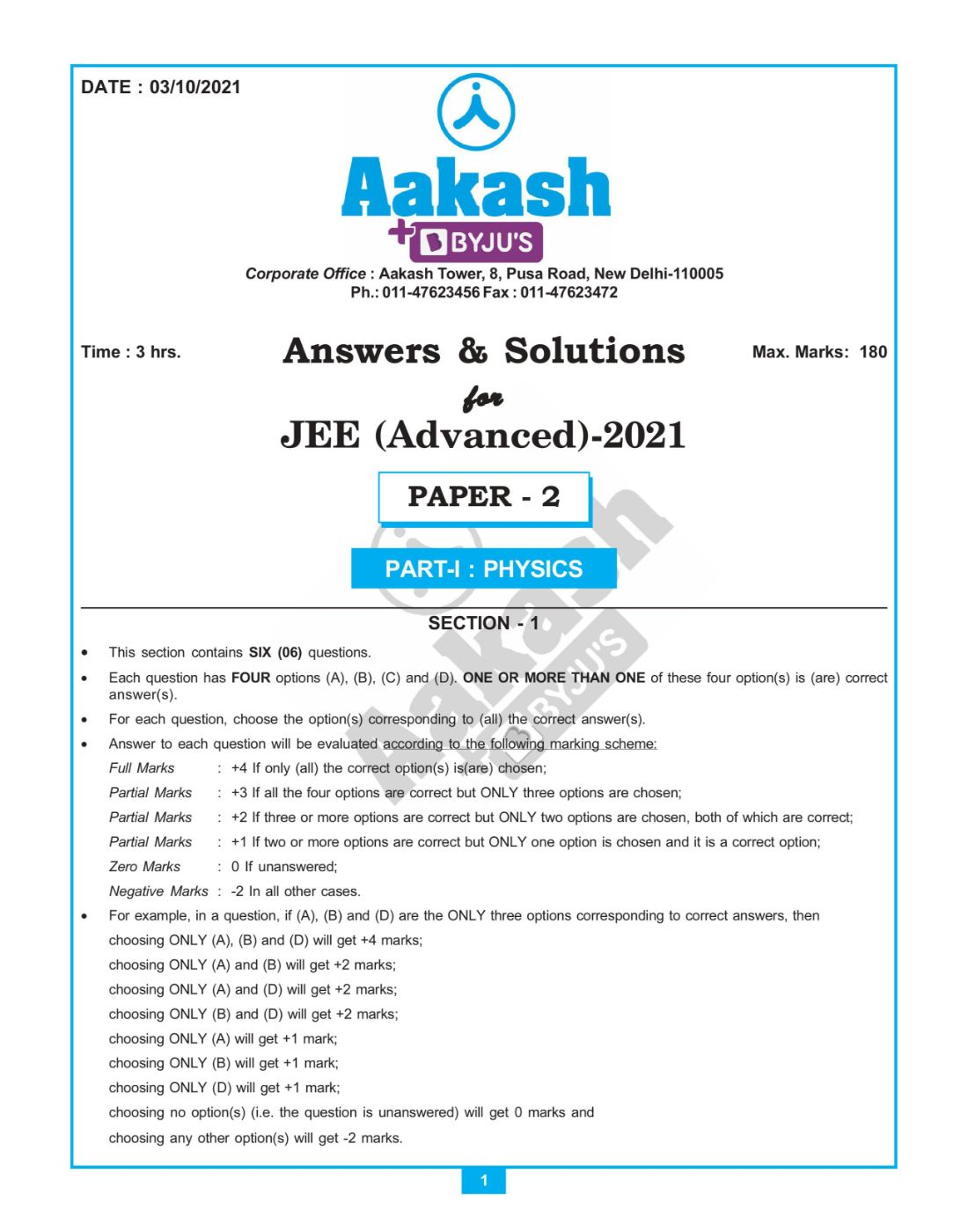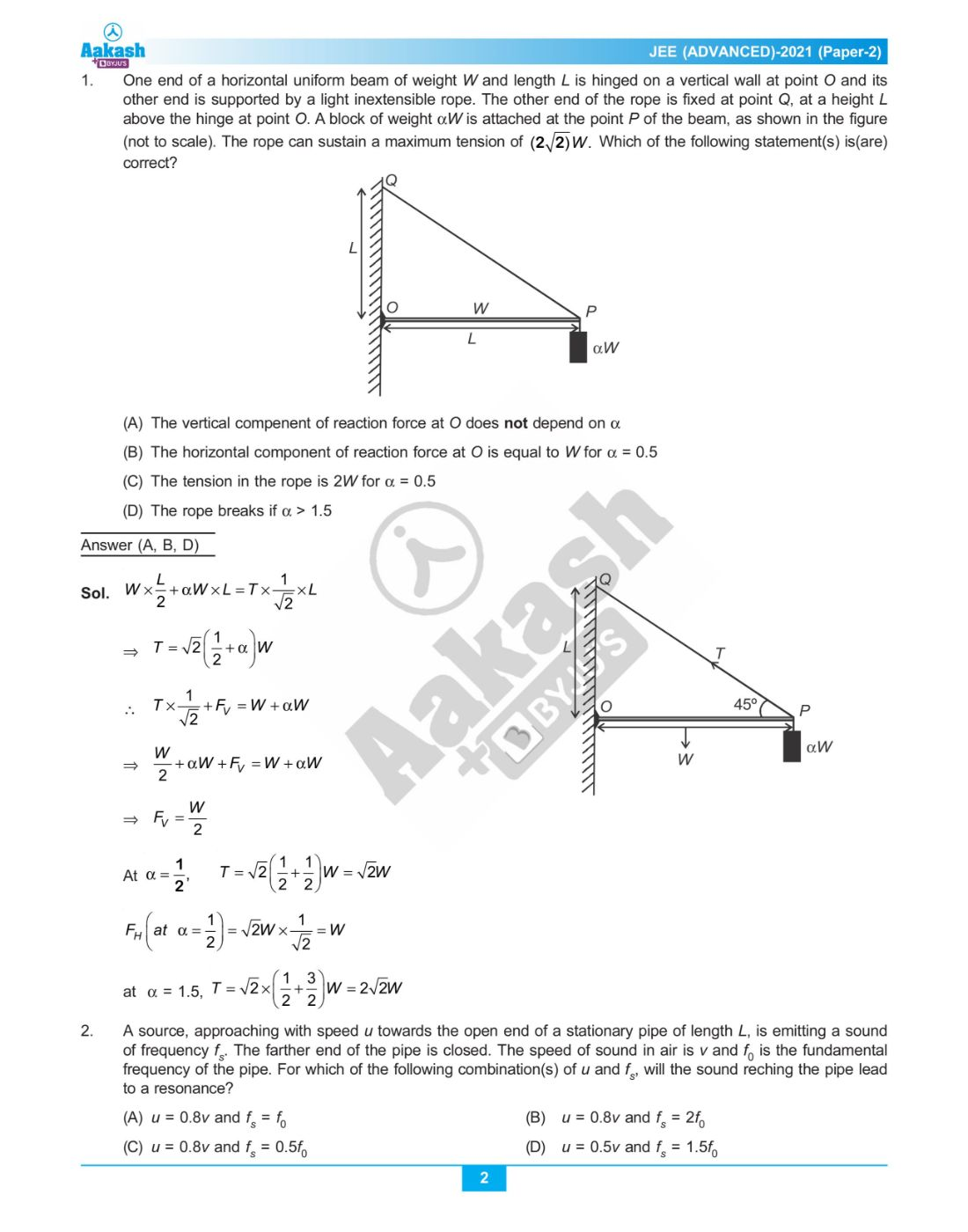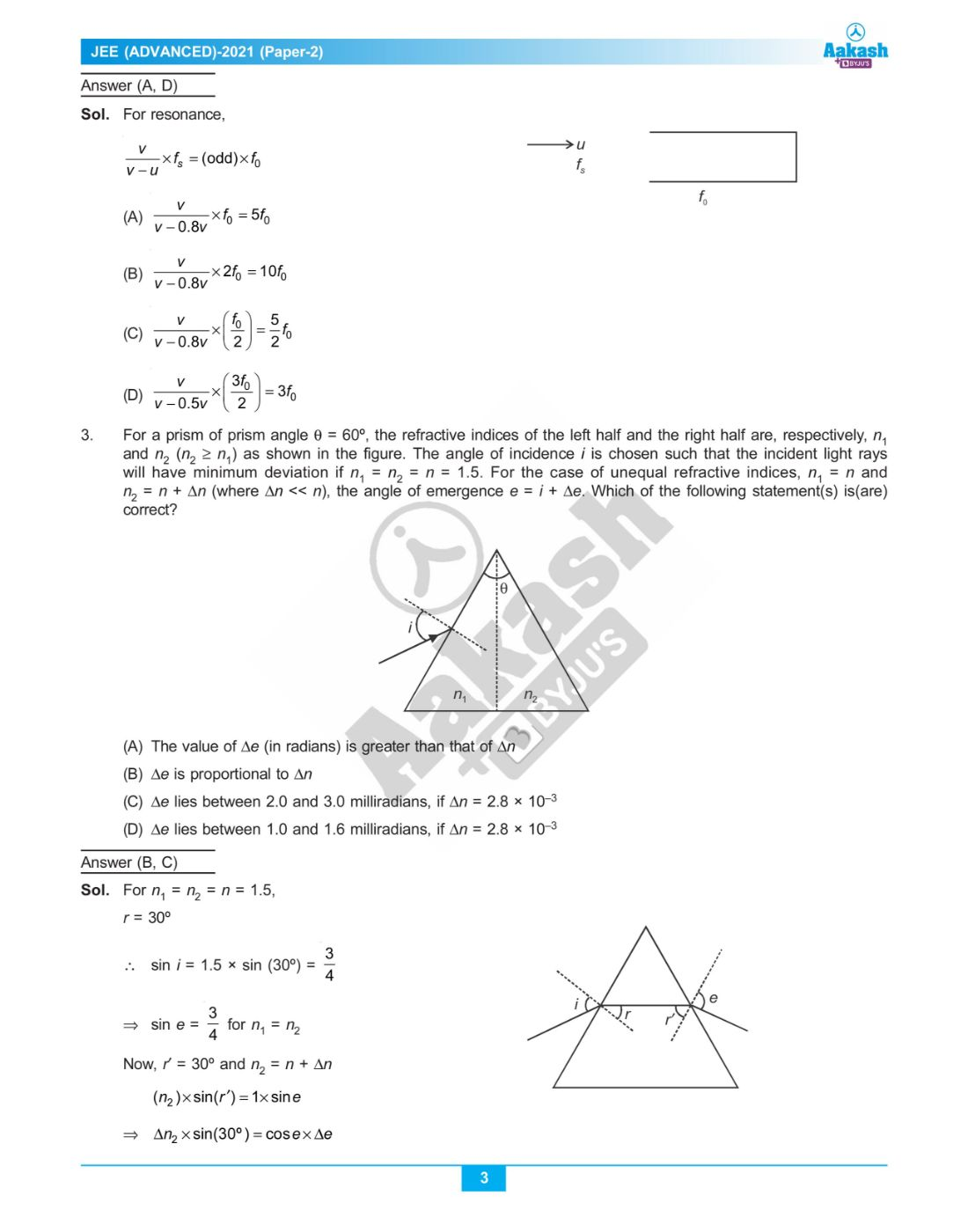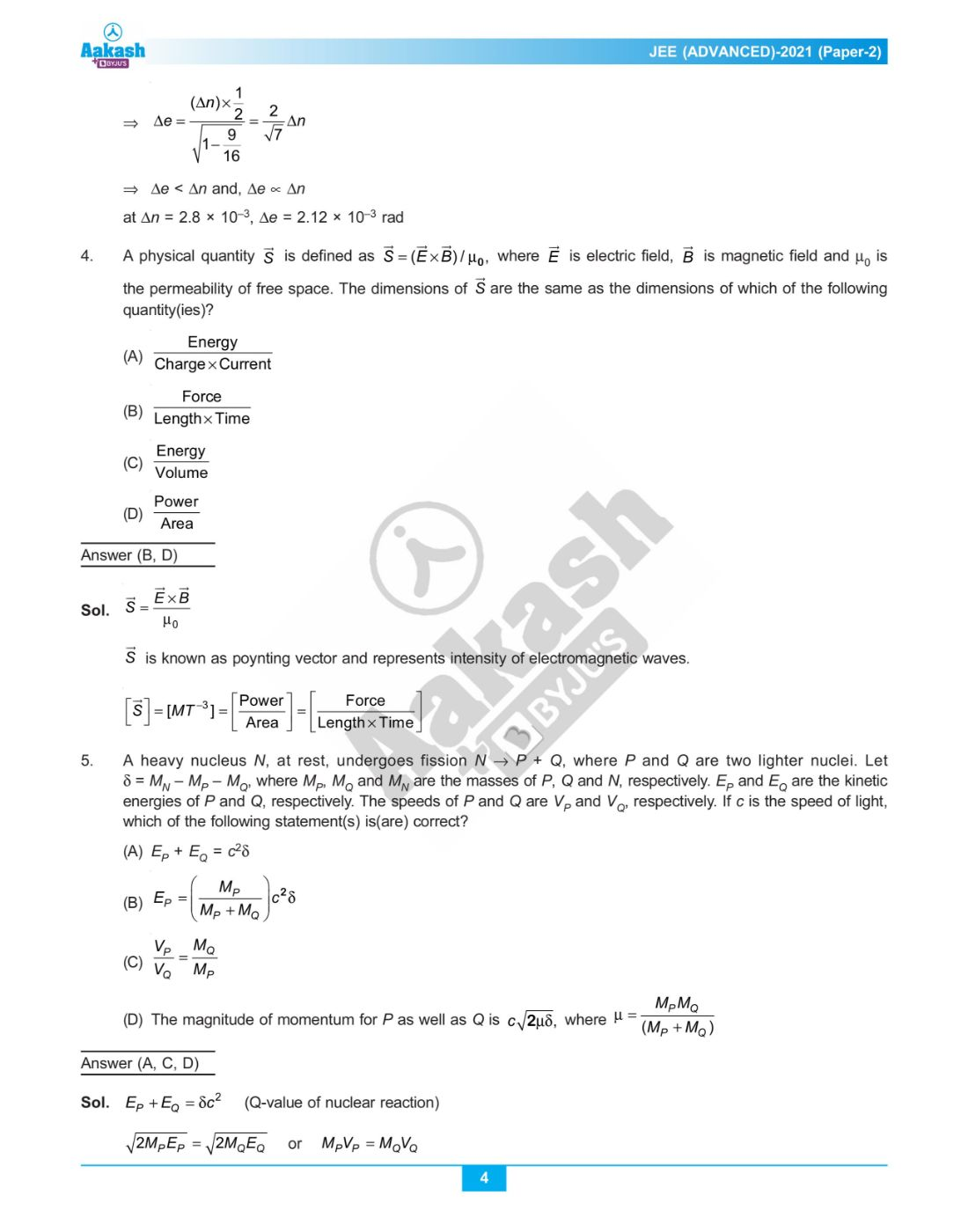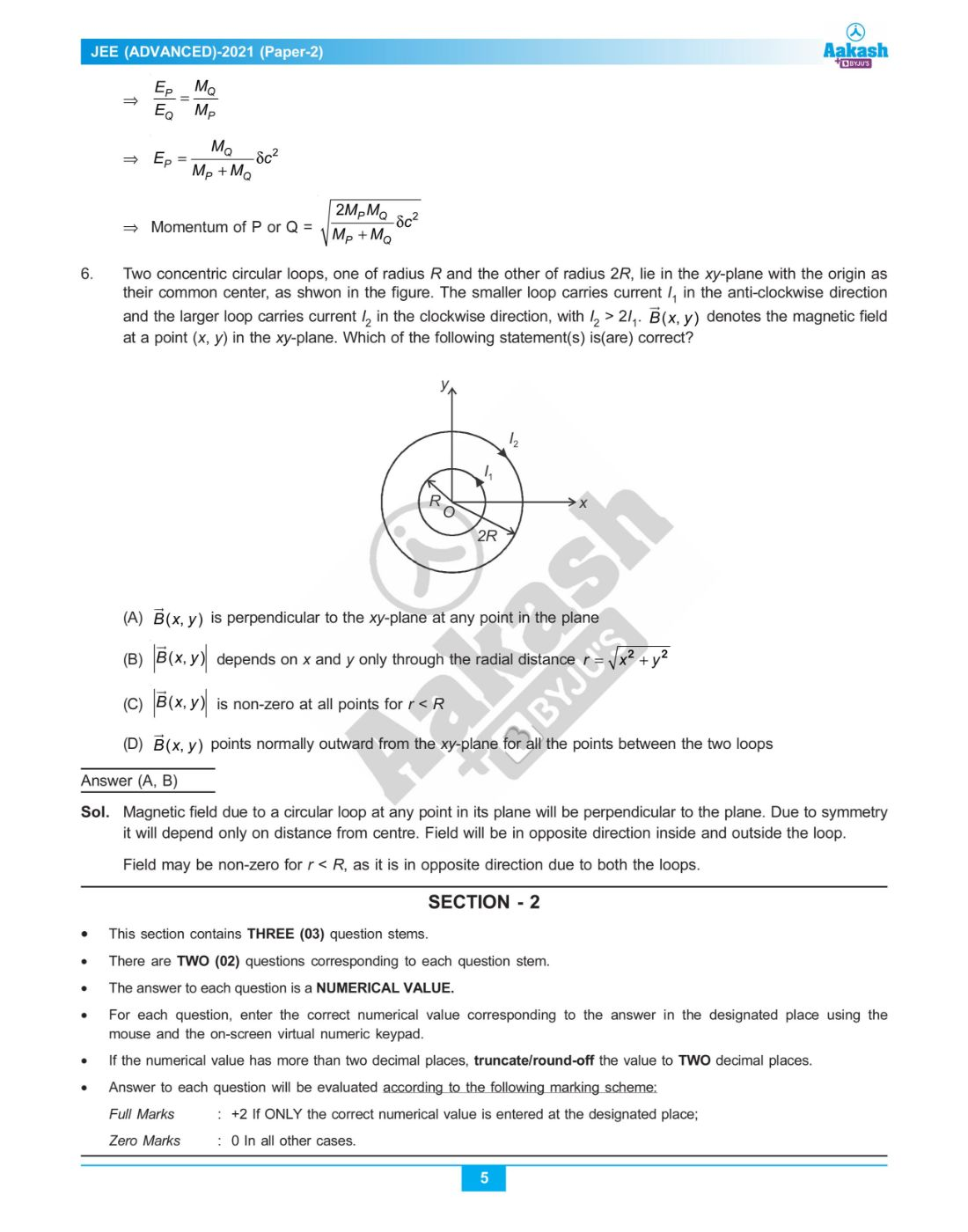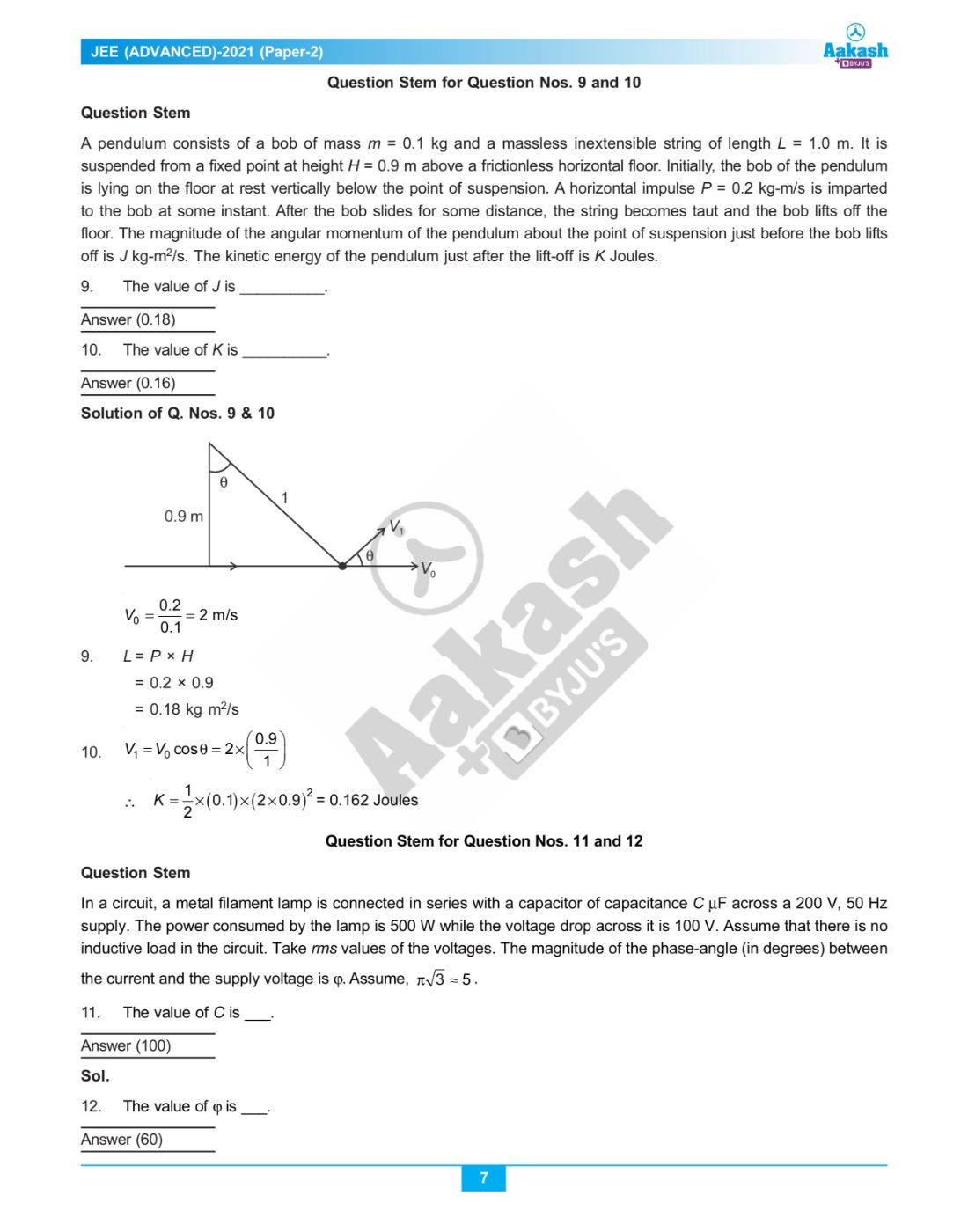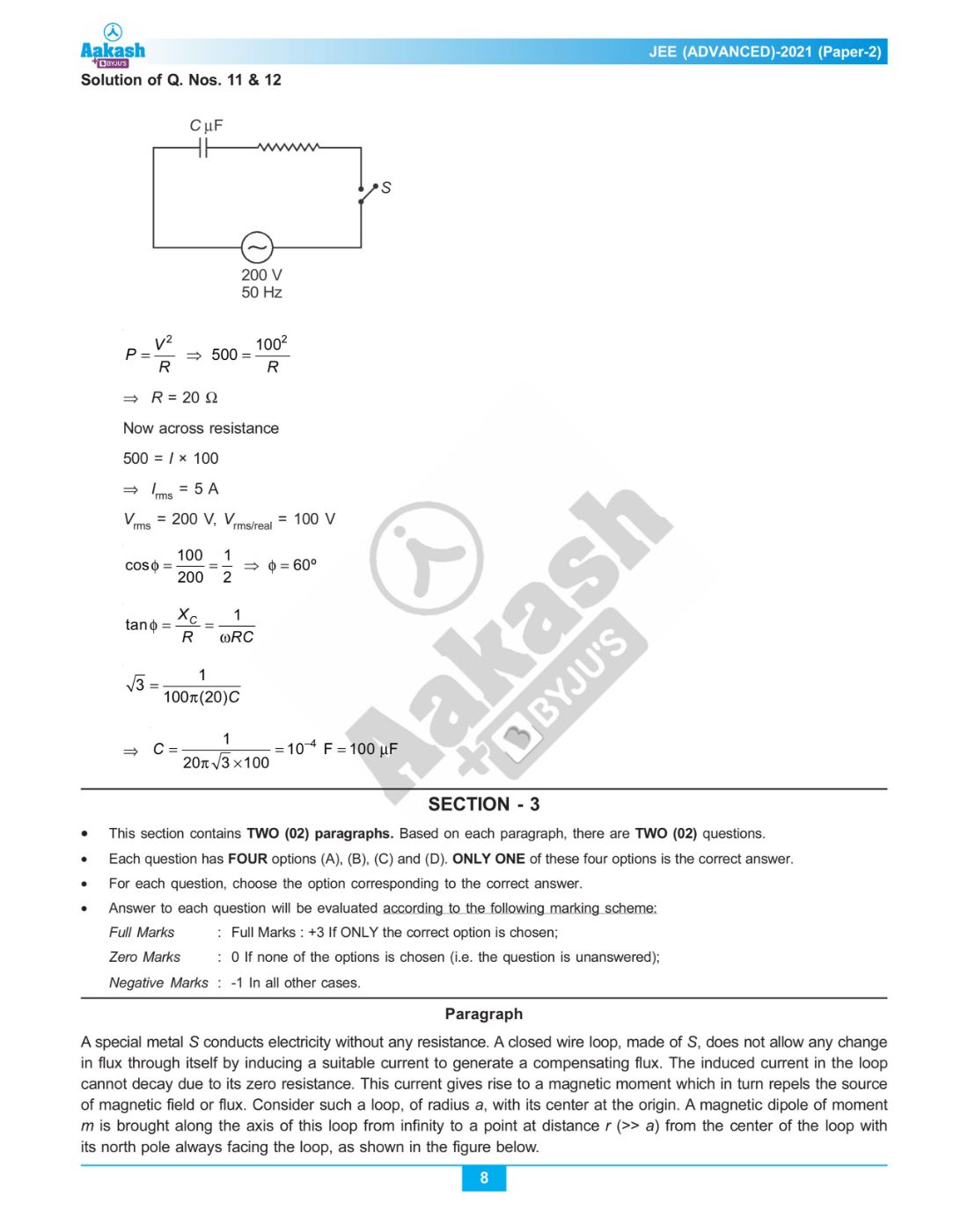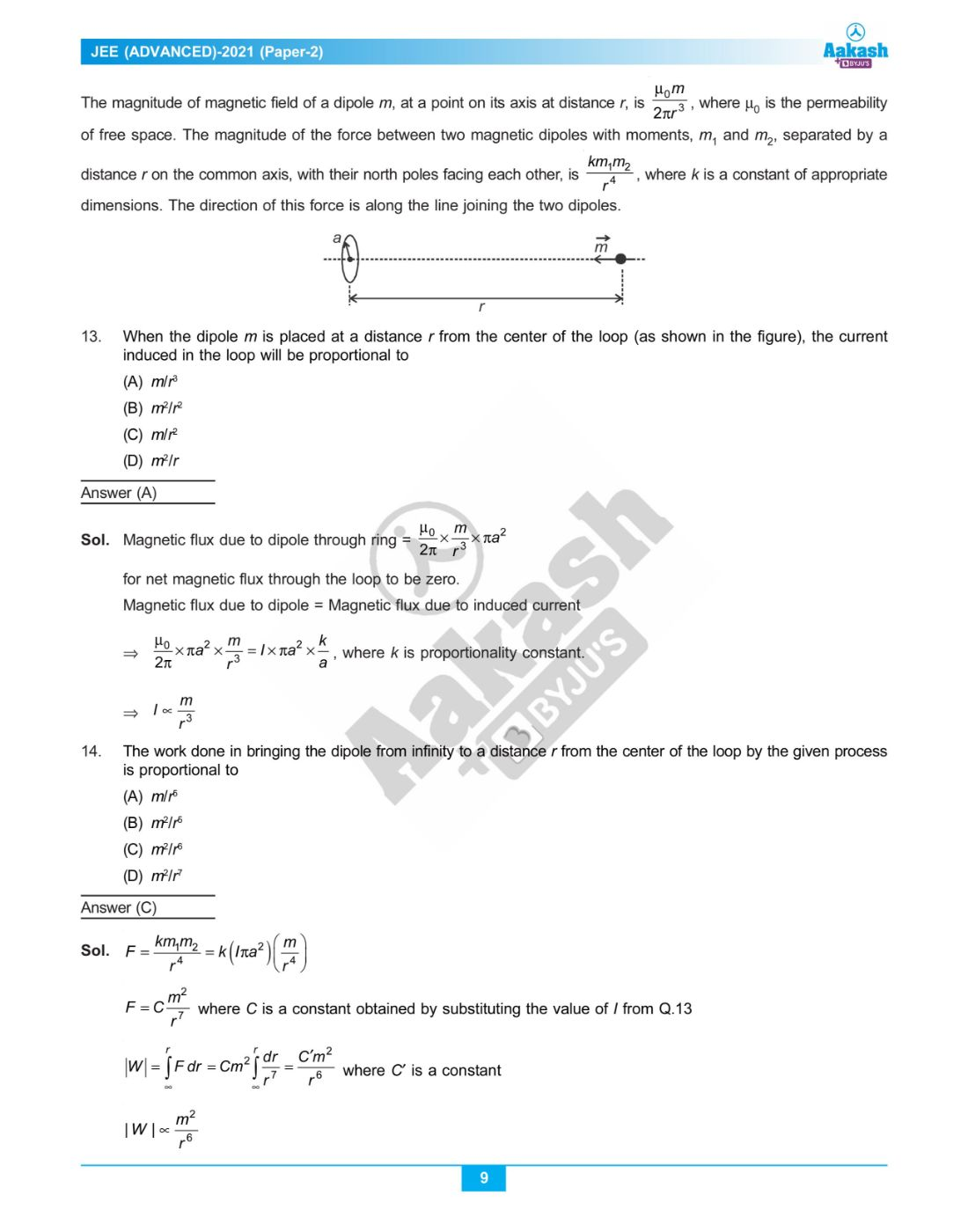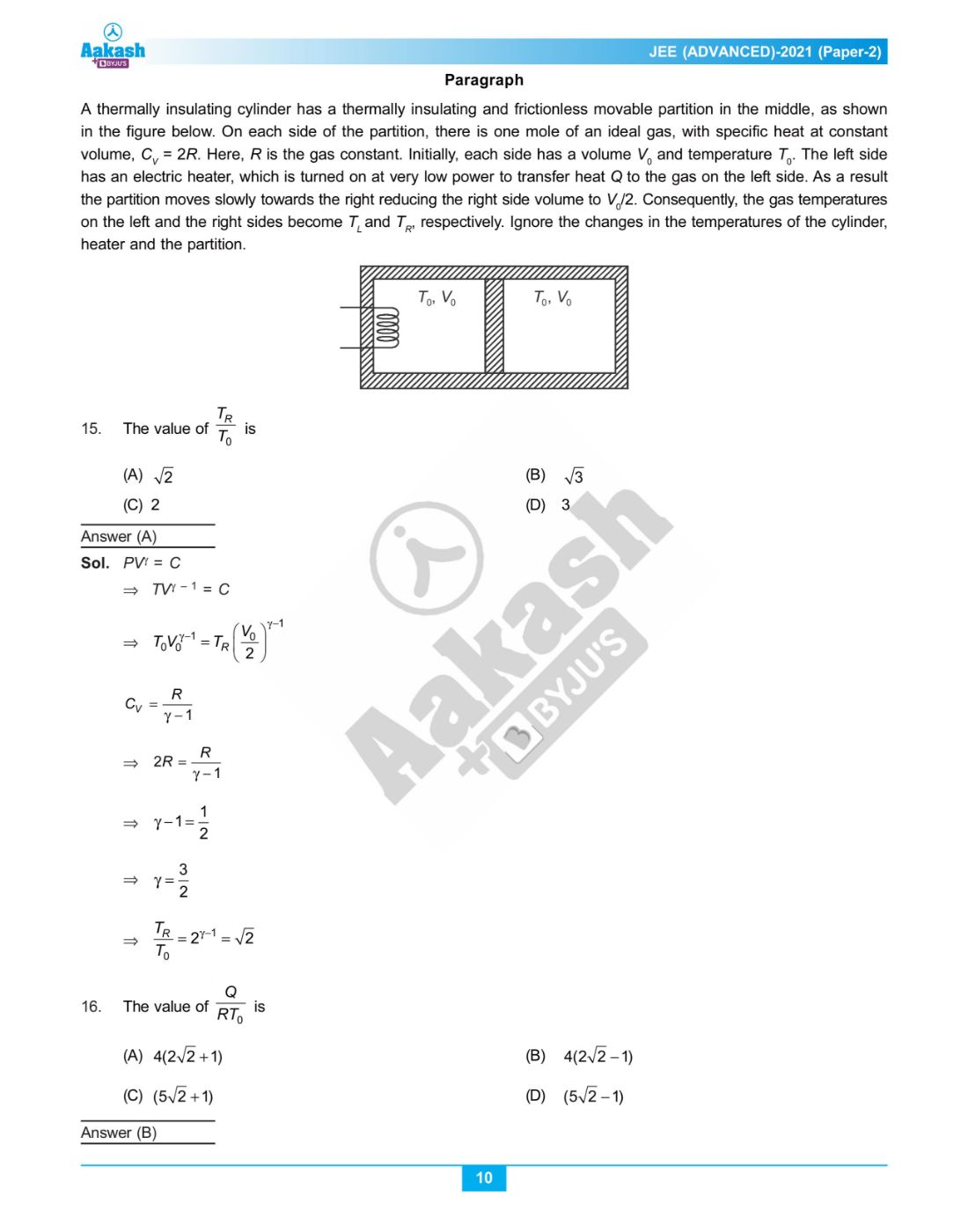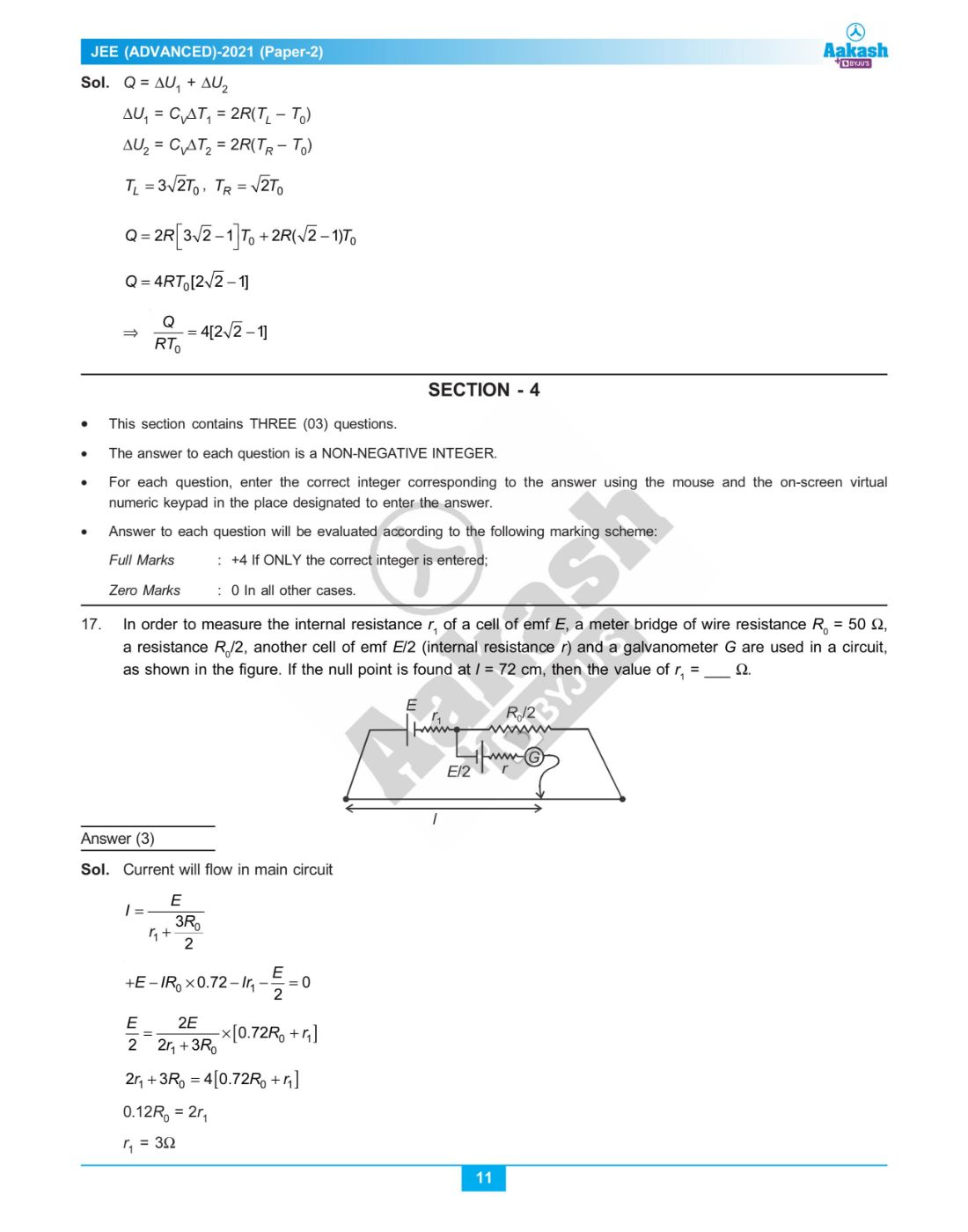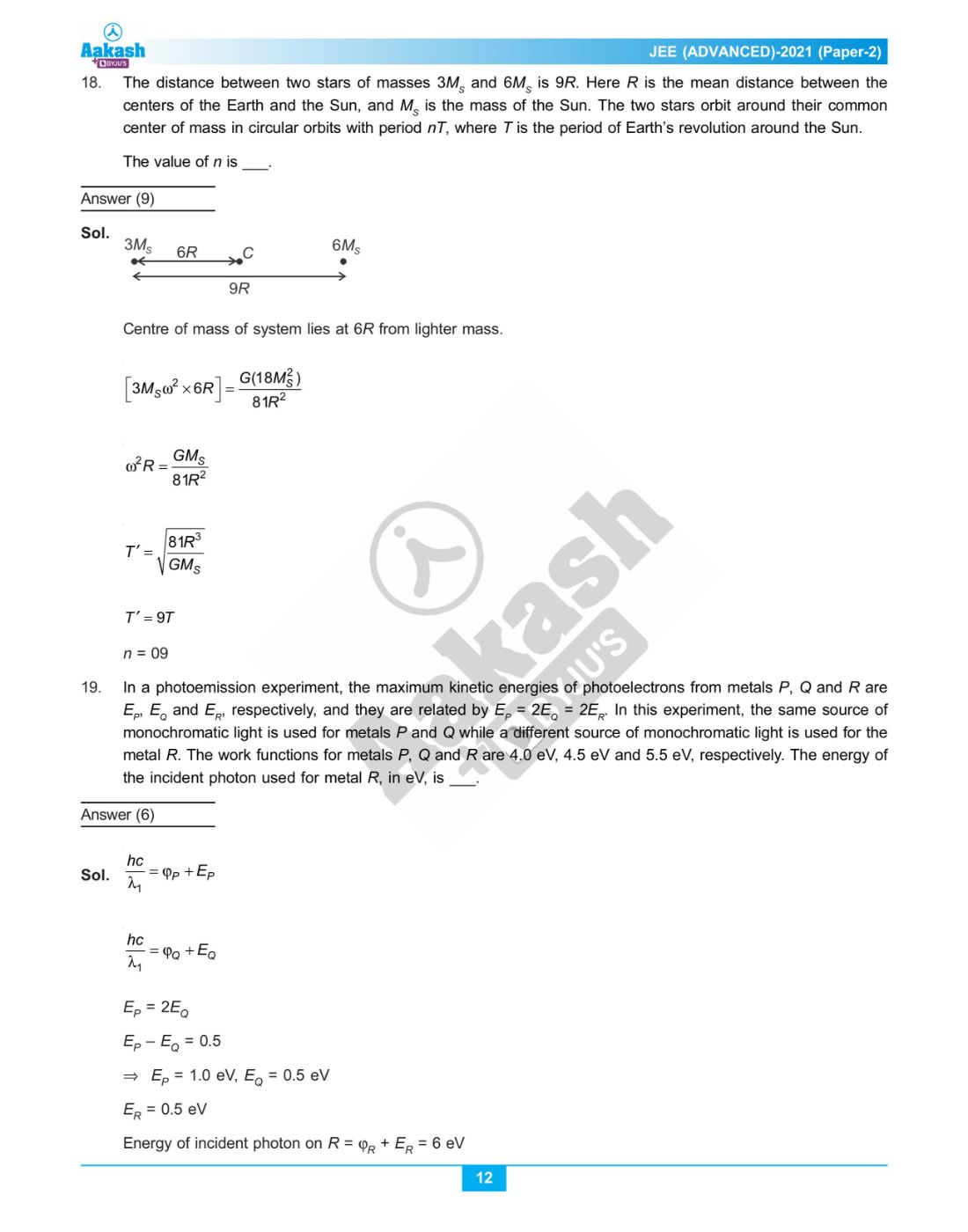Know more about the Repeaters Batch Know more about the Repeaters Batch

# JEE Advanced Question Paper 2021 Physics Paper 2

JEE Advanced 2021 Paper 2 Physics Question Paper

Question 1: One end of a horizontal uniform beam of weight W and length L is hinged on a vertical wall at point O and its other end is supported by a light inextensible rope. The other end of the rope is fixed at point Q, at a height L above the hinge at point O. A block of weight αW is attached at point P of the beam, as shown in the figure (not to scale). The rope can sustain a maximum tension of (2√2) W Which of the following statement(s) is(are) correct?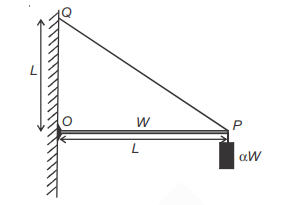a. The vertical component of the reaction force at O does not depend on α
b. The horizontal component of the reaction force at O is equal to W for α = 0.5
c. The tension in the rope is 2W for α = 0.5
d. The rope breaks if α > 1.5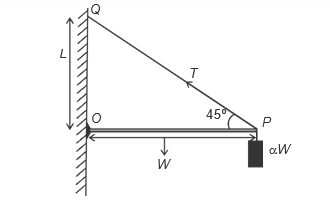W x (L/2) + αW x L = T x (1/√2) x L

$$\begin{array}{l}\Rightarrow T = \sqrt{2}\left ( \frac{1}{2}+\alpha \right )W\end{array}$$
$$\begin{array}{l}T\times \frac{1}{\sqrt{2}}+F_{v}= W+\alpha W\end{array}$$
$$\begin{array}{l}\frac{W}{2}+\alpha W+F_{v}= W+\alpha W\end{array}$$

Fv = W/2

At α = ½,

$$\begin{array}{l}T = \sqrt{2}\left ( \frac{1}{2}+\frac{1}{2} \right )W\end{array}$$
= √2W

FH (at α = ½) = √2W x ½ = W

At α = 1.5, T = √2 x (½ + 3/2) W = 2√2W

Question 2: A source, approaching with speed u towards the open end of a stationary pipe of length L, is emitting a sound of frequency fs. The farther end of the pipe is closed. The speed of sound in air is v and f0 is the fundamental frequency of the pipe. For which of the following combination(s) of u and f s, will the sound reaching the pipe lead to a resonance?

a. u = 0.8v and fs = f0
b. u = 0.8v and fs = 2f0
c. u = 0.8v and fs = 0.5f0
d. u = 0.5v and fs = 1.5f0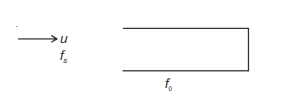$$\begin{array}{l}\frac{v}{v-u}\times f_{s}= (odd)\times f_{0}\end{array}$$

(a)

$$\begin{array}{l}\frac{v}{v-0.8v}\times f_{0}= 5 f_{0}\end{array}$$

(b)

$$\begin{array}{l}\frac{v}{v-0.8v}\times 2f_{0}= 10 f_{0}\end{array}$$

(c)

$$\begin{array}{l}\frac{v}{v-0.8v}\times \frac{f_{0}}{2}= (5/2)f_{0}\end{array}$$

(d)

$$\begin{array}{l}\frac{v}{v-0.5v}\times \frac{3f_{0}}{2}= 3f_{0}\end{array}$$

Question 3: For a prism of prism angle θ = 60º, the refractive indices of the left half and the right half are, respectively, n1 and n2 (n2 ≥ n1) as shown in the figure. The angle of incidence is chosen such that the incident light rays will have minimum deviation if n1 = n2 = n = 1.5. For the case of unequal refractive indices, n1 = n and n2 = n + Δn (where Δn << n), the angle of emergence e = i + Δe. Which of the following statement(s) is(are) correct?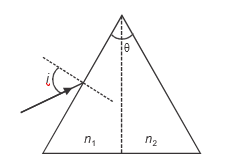a. The value of Δe (in radians) is greater than that of Δn
b. Δe is proportional to Δn
c. Δe lies between 2.0 and 3.0 milliradians if Δn = 2.8 × 10–3
d. Δe lies between 1.0 and 1.6 milliradians if Δn = 2.8 × 10–3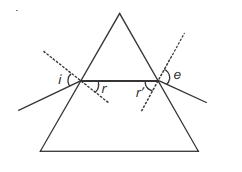For n1 = n2 = n = 1.5,

r = 30º

Therefore, sin i = 1.5 × sin (30º) = 3/4

⇒ sin e = 3/4 for n1 = n2

Now, r′ = 30º and n2 = n + Δn

n2 x sin (r’) = 1 x sin e

⇒ Δn2 x sin 300= cos e x Δe

$$\begin{array}{l}\Rightarrow \Delta e = \frac{(\Delta n)\times \frac{1}{2}}{\sqrt{1-\frac{9}{16}}}= \frac{2}{\sqrt{7}}\Delta n\end{array}$$

⇒ Δe < Δn and, Δe ∝ Δn

At Δn = 2.8 × 10–3, Δe = 2.12 × 10–3 rad

Question 4: A physical quantity

$$\begin{array}{l}\vec{S}\end{array}$$
is defined as
$$\begin{array}{l}\vec{S} = (\vec{E}\times \vec{B})/\mu _{0}\end{array}$$
, where
$$\begin{array}{l}\vec{E}\end{array}$$
is electric field,
$$\begin{array}{l}\vec{B}\end{array}$$
is magnetic field and μ0 is the permeability of free space. The dimensions of
$$\begin{array}{l}\vec{S}\end{array}$$
are the same as the dimensions of which of the following quantity(ies)?

a. Energy /(Charge x Current)
b. Force/ (Length x Time)
c. Energy/Volume
d. Power/Area

$$\begin{array}{l}\vec{S} = (\vec{E}\times \vec{B})/\mu _{0}\end{array}$$
$$\begin{array}{l}\vec{S}\end{array}$$
is known as poynting vector and represents intensity of electromagnetic waves

$$\begin{array}{l}\left [ \vec{S} \right ]=\left [ MT^{-3} \right ]=\left [ \frac{Power}{Area} \right ]= \left [ \frac{Force}{Length \times Time} \right ]\end{array}$$

Question 5: A heavy nucleus N, at rest, undergoes fission N → P + Q, where P and Q are two lighter nuclei. Let δ = MN – MP – MQ, where MP, MQ and MN are the masses of P, Q and N, respectively. EP and EQ are the kinetic energies of P and Q, respectively. The speeds of P and Q are VP and VQ, respectively. If c is the speed of light, which of the following statement(s) is(are) correct?

a. EP + EQ = c2δ
b.

$$\begin{array}{l}E_{p}=\left ( \frac{M_{P}}{M_{P}+M_{Q}} \right )c^{2}\delta\end{array}$$

c.
$$\begin{array}{l}\frac{V_{P}}{V_{Q}}=\frac{M_{Q}}{M_{P}}\end{array}$$

d. The magnitude of momentum for P as well as Q is
$$\begin{array}{l}c\sqrt{2\mu\delta }\end{array}$$
, where
$$\begin{array}{l}\mu =\frac{M_{P}M_{Q}}{M_{P}+M_{Q}}\end{array}$$

EP +EQ = δc2 (Q-value of nuclear reaction)

$$\begin{array}{l}\sqrt{2M_{P}E_{P}}=\sqrt{2M_{Q}E_{Q}}\end{array}$$

MPVP = MQVQ

$$\begin{array}{l}\Rightarrow \frac{E_{P}}{E_{Q}}=\frac{M_{Q}}{M_{P}}\end{array}$$
$$\begin{array}{l}\Rightarrow E_{P}= \frac{M_{Q}}{M_{P}+M_{Q}}\delta c^{2}\end{array}$$

⇒ Momentum of P or

$$\begin{array}{l}Q = \sqrt{\frac{2M_{P}M_{Q}}{M_{P}+M_{Q}}\delta c^{2}}\end{array}$$

Question 6: Two concentric circular loops, one of radius R and the other of radius 2R lie in the xy-plane with the origin as their common centre, as shown in the figure. The smaller loop carries current I1 in the anti-clockwise direction and the larger loop carries current I2 in the clockwise direction, with I2 > 2I1.

$$\begin{array}{l}\vec{B}(x,y)\end{array}$$
denotes the magnetic field at a point (x, y) in the xy-plane. Which of the following statement(s) is(are) correct?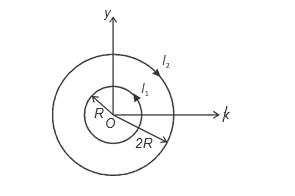a.

$$\begin{array}{l}\vec{B}(x,y)\end{array}$$
is perpendicular to the xy-plane at any point in the plane

b.
$$\begin{array}{l}\left | \vec{B}(x,y) \right |\end{array}$$
depends on x and y only through the radial distance
$$\begin{array}{l}r =\sqrt{x^{2}+y^{2}}\end{array}$$

c.
$$\begin{array}{l}\left | \vec{B}(x,y) \right |\end{array}$$
is non-zero at all points for r < R

d.
$$\begin{array}{l}\vec{B}(x,y)\end{array}$$
points normally outward from the xy-plane for all the points between the two loops

A magnetic field due to a circular loop at any point in its plane will be perpendicular to the plane. Due to symmetry, it will depend only on the distance from the centre. The field will be in opposite direction inside and outside the loop. The field may be non-zero for r < R, as it is in opposite direction due to both the loops.

Question Stem for Question Nos. 7 and 8

A soft plastic bottle, filled with water of density 1 gm/cc, carries an inverted glass test tube with some air (ideal gas) trapped as shown in the figure. The test tube has a mass of 5 gm, and it is made of a thick glass of density 2.5 gm/cc. Initially, the bottle is sealed at atmospheric pressure p0 = 105 Pa so that the volume of the trapped air is V0 = 3.3 cc. When the bottle is squeezed from outside at a constant temperature, the pressure inside rises and the volume of the trapped air reduces. It is found that the test tube begins to sink at pressure p0 + Δp without changing its orientation. At this pressure, the volume of the trapped air is V0 – ΔV.

Let ΔV = X cc and Δp = Y × 103 Pa.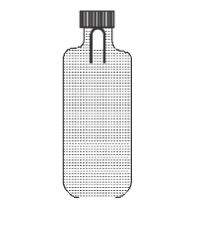Question 7: The value of X is __________.

Question 8: The value of Y is __________.

Solution of Q. Nos. 7 & 8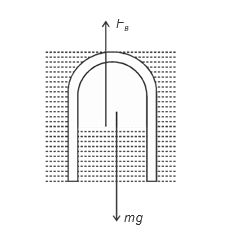When buoyant force on (tube + air) system will become equal to the weight of the tube then the tube will start sinking. (Here we can neglect weight of air as compared to weight of tube)

Now, Let volume of air in this case = Vair

FB = mg

So, δw (Vtube + Vair) g = mg

$$\begin{array}{l}1\left ( \frac{5}{2.5}cm^{3}+V_{air} \right ) =5\end{array}$$

⇒ 2 + Vair = 5

Vair = 3 cm3

As initial volume of air = 3.3 cm3

So, ΔV = 0.3 cc

So, X = 0.30

As temperature of air is constant

So, PV = constant

P0 3.3 = Pf 3, Pf is final pressure of air

⇒ Pf = 1.1 P0 = P0 + 0.1 P0

So, ΔP = 104 Pa

So, Y = 10

So, X = 0.30

Y = 10.00

Question Stem for Question Nos. 9 and 10

A pendulum consists of a bob of mass m = 0.1 kg and a massless inextensible string of length L = 1.0 m. It is suspended from a fixed point at height H = 0.9 m above a frictionless horizontal floor. Initially, the bob of the pendulum is lying on the floor at rest vertically below the point of suspension. A horizontal impulse P = 0.2 kg-m/s is imparted to the bob at some instant. After the bob slides for some distance, the string becomes taut and the bob lifts off the floor. The magnitude of the angular momentum of the pendulum about the point of suspension just before the bob lifts off is J kg-m2/s. The kinetic energy of the pendulum just after the lift-off is K Joules.

Question 9: The value of J is __________.

Question 10: The value of K is __________.

Solution of Q. Nos. 9 and 10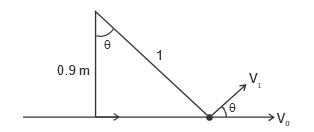V0 =0.2/0.1 =2 m/s

9. L = P × H

= 0.2 × 0.9 = 0.18 kg m2/s

10. V1=V0 cos θ = 2 x (0.9/1)

K = (½) x (0.1) x (2 x 0.9)2

= 0.162 Joules

Question Stem for Question Nos. 11 and 12

In a circuit, a metal filament lamp is connected in series with a capacitor of capacitance C μF across a 200 V, 50 Hz supply. The power consumed by the lamp is 500 W while the voltage drop across it is 100 V. Assume that there is no inductive load in the circuit. Take rms values of the voltages. The magnitude of the phase angle (in degrees) between the current and the supply voltage is ϕ. Assume, π√3 = 5.

Question 11: The value of C is ___.

Question 12: The value of ϕ is ___.

Solution of Q. Nos. 11 & 12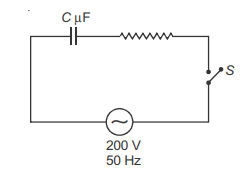P = V2/2

⇒ 500 = 1002/R

⇒ R = 20 Ω

Now across resistance 500 = I × 100

⇒ I rms = 5 A

Vrms = 200 V,

Vrms/real = 100 V

cos φ = 100/200 = ½ ⇒ Φ = 600

tan Φ = XC/ R = 1/ωRC

√3 = 1/100π(20)C

C = 1/(20π√3 x 100)

= 10-4 F

= 100 μF

Question Stem for Question Nos. 13 and 14

A special metal S conducts electricity without any resistance. A closed wire loop, made of S, does not allow any change in flux through itself by inducing a suitable current to generate a compensating flux. The induced current in the loop cannot decay due to its zero resistance. This current gives rise to a magnetic moment which in turn repels the source of magnetic field or flux. Consider such a loop, of radius a, with its centre at the origin. A magnetic dipole of moment m is brought along the axis of this loop from infinity to a point at distance r (>> a) from the centre of the loop with its north pole always facing the loop, as shown in the figure below.

The magnitude of the magnetic field of a dipole m, at a point on its axis at distance r, is

$$\begin{array}{l}\frac{\mu _{0}m}{2\Pi r^{3}}\end{array}$$
, where μ0 is the permeability of free space. The magnitude of the force between two magnetic dipoles with moments, m1 and m2, separated by a distance r on the common axis, with their north poles facing each other, is km1m2/r4, where k is a constant of appropriate dimensions. The direction of this force is along the line joining the two dipoles.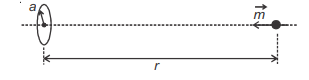Question 13: When the dipole m is placed at a distance r from the centre of the loop (as shown in the figure), the current induced in the loop will be proportional to?

a. m/r3
b. m2 /r2
c. m/r2
d. m2 /r

Magnetic flux due to dipole through ring =

$$\begin{array}{l}\frac{\mu _{0}}{2\Pi }\times \frac{m}{r^{3}}\times \Pi a^{2}\end{array}$$
for net magnetic flux through the loop to be zero.

Magnetic flux due to dipole = Magnetic flux due to induced current

$$\begin{array}{l}\frac{\mu _{0}}{2\Pi }\times \Pi a^{2}\times \frac{m}{r^{3}}=l\times \Pi a^{2}\times \frac{k}{a}\end{array}$$
, where k is proportionality constant.

⇒ l∝ m/r3

Question 14: The work done in bringing the dipole from infinity to a distance r from the centre of the loop by the given process is proportional to?

a. m/r5
b. m2 /r5
c. m2 /r6
d. m2 /r7

$$\begin{array}{l}F = \frac{km_{1}m_{2}}{r^{4}}=k(l\pi a^{2})\left ( \frac{m}{r^{4}} \right )\end{array}$$

F = C(m2/r7) where C is a constant obtained by substituting the value of I from Q.13

$$\begin{array}{l}\left | W \right |=\int_{\infty }^{r}Fdr=Cm^{2}\int_{\infty }^{r}\frac{dr}{r^{7}}=\frac{C’m^{2}}{r^{6}}\end{array}$$
where C′ is a constant

$$\begin{array}{l}\left | W \right |\propto \frac{m^{2}}{r^{6}}\end{array}$$

Question Stem for Question Nos. 15 and 16

A thermally insulating cylinder has a thermally insulating and frictionless movable partition in the middle, as shown in the figure below. On each side of the partition, there is one mole of an ideal gas, with specific heat at constant volume, CV = 2R. Here, R is the gas constant. Initially, each side has a volume V0 and temperature T0. The left side has an electric heater, which is turned on at very low power to transfer heat Q to the gas on the left side. As a result, the partition moves slowly towards the right, reducing the right side volume to V0 /2. Consequently, the gas temperatures on the left and the right sides become TL and TR, respectively. Ignore the changes in the temperatures of the cylinder, heater and partition.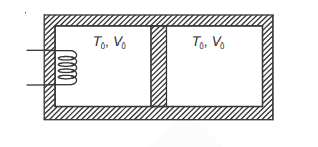Question 15: The value of TR/T0 is

a. √2
b. √3
c. 2
d. 3

PVץ = C

$$\begin{array}{l}TV^{\gamma -1}=C\end{array}$$
$$\begin{array}{l}\Rightarrow T_{0}V_{0}^{\gamma -1}= T_{R}(\frac{V_{0}}{2})^{\gamma -1}\end{array}$$
$$\begin{array}{l}C_{V}=\frac{R}{\gamma -1}\end{array}$$
$$\begin{array}{l}\Rightarrow 2R=\frac{R}{\gamma -1}\end{array}$$
$$\begin{array}{l}\gamma -1=\frac{1}{2}\end{array}$$
$$\begin{array}{l}\gamma=\frac{3}{2}\end{array}$$
$$\begin{array}{l}\Rightarrow \frac{T_{R}}{T_{0}}=2^{\gamma -1}=\sqrt{2}\end{array}$$

Question 16: The value of Q/RT0 is

a. 4(2√2 +1)
b. 4(2√2 -1)
c. (5√2 +1)
d. (5√2 -1)

Q = ΔU1 + ΔU2

ΔU1 = CVΔT1 = 2R(TL – T0)

ΔU2 = CVΔT2 = 2R(TR – T0)

T L = 3√2T0 , TR = √2T0

Q = 2R[3√2 -1]T0+ 2R(√2 -1]T0

Q = 4RT0[2√2 -1]

⇒ Q/RT0 = 4[2√2 -1]

Question 17:In order to measure the internal resistance r1 of a cell of emf E, a meter bridge of wire resistance R0 = 50 Ω, a resistance R0/2, another cell of emf E/2 (internal resistance r) and a galvanometer G are used in a circuit, as shown in the figure. If the null point is found at l = 72 cm, then the value of r 1 = ___ Ω.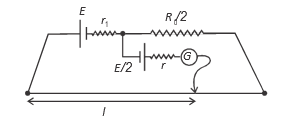Current will flow in the main circuit

$$\begin{array}{l}I = \frac{E}{r_{1}+\frac{3R_{0}}{2}}\end{array}$$
$$\begin{array}{l}+E- IR_{0}\times 0.72-Ir_{1}-\frac{E}{2}=0\end{array}$$
$$\begin{array}{l}\frac{E}{2}=\frac{2E}{2r_{1}+3R_{0}}\times [0.72R_{0}+r_{1}]\end{array}$$
$$\begin{array}{l}2r_{1}+3R_{0}= 4[0.72R_{0}+r_{1}]\end{array}$$

0.12R0 = 2r1

r1 = 3Ω

Question 18:The distance between two stars of masses 3MS and 6MS is 9R. Here R is the mean distance between the centres of the Earth and the Sun, and MS is the mass of the Sun. The two stars orbit around their common centre of mass in circular orbits with period nT, where T is the period of Earth’s revolution around the Sun. The value of n is ___.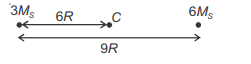Centre of mass of system lies at 6R from lighter mass

$$\begin{array}{l}\left [ 3M_{s}\omega ^{2}\times 6R \right ]=\frac{G(18M^{2}_{s})}{81R^{2}}\end{array}$$
$$\begin{array}{l}\omega ^{2}R = \frac{GM}{81R^{2}}\end{array}$$
$$\begin{array}{l}T^{‘}=\sqrt{\frac{81R^{3}}{GM_{s}}}\end{array}$$

T’ = 9T

n = 09

Question 19: In a photoemission experiment, the maximum kinetic energies of photoelectrons from metals P, Q and R are EP, EQ and ER, respectively, and they are related by EP = 2EQ = 2ER. In this experiment, the same source of monochromatic light is used for metals P and Q while a different source of monochromatic light is used for metal R. The work functions for metals P, Q and R are 4.0 eV, 4.5 eV and 5.5 eV, respectively. The energy of the incident photon used for metal R, in eV, is ___.

$$\begin{array}{l}\frac{hc}{\lambda _{1}}=\phi _{p}+E_{p}\end{array}$$
$$\begin{array}{l}\frac{hc}{\lambda _{1}}=\phi _{Q}+E_{Q}\end{array}$$

Ep = 2EQ

EP– EQ = 0.5

⇒ Ep = 1.0 eV, EQ = 0.5 eV

ER = 0.5 eV

Energy of incident photon on R = φR + ER = 6 eV